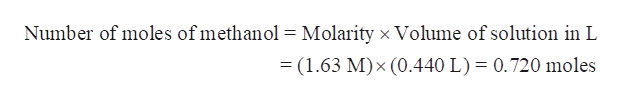# How many grams of methanol (CH3OH, FM 32.04) are contained in 0.440 L of 1.63 M aqueous methanol (i.e., 1.63 mol CH3OH/L solution)?

Question
12 views

How many grams of methanol (CH3OH, FM 32.04) are contained in 0.440 L of 1.63 M aqueous methanol (i.e., 1.63 mol CH3OH/L solution)?

check_circle

Step 1

Step 1-

We have to calculate the mass of methanol in grams that are contained in 0.440 L of 1.63 M aqueous methanol.

Thus, V = 0.440 L

Molarity = 1.63 M

Molar mass of methanol = 32.04 g/mol

Step 2

Step 2-

Molarity is a term used to express the concentration of a solution and is defined as the number of moles of solute per litre of solution.

Step 3

Step 3-Calculate the moles of methanol...help_outlineImage TranscriptioncloseNumber of moles of methanol = Molarity x Volume of solution in L = (1.63 M) x (0.440 L) 0.720 moles fullscreen

### Want to see the full answer?

See Solution

#### Want to see this answer and more?

Solutions are written by subject experts who are available 24/7. Questions are typically answered within 1 hour.*

See Solution
*Response times may vary by subject and question.
Tagged in

### Chemistry